## Artigo

•Citado por SciELO
•Acessos

•Similares em SciELO

## versão impressa ISSN 1665-2738

### Rev. Mex. Ing. Quím vol.10 no.3 Ciudad de México Dez. 2011

Ingeniería de procesos

Grey heat integration

Integración de calor gris

E.D. Gálvez1,2, M.E. Mellado1, P.S. Patiño3, K.L. Ossandón3 and L.C. Cisternas1,3*

1 Centro de Investigación Científico Tecnológico para la Minería. Antofagasta, Chile.

2 Universidad Católica del Norte. Antofagasta, Chile.

3 Universidad de Antofagasta. Antofagasta, Chile. *Corresponding author. E–mail: lcisternas@uantof.cl Tel. 56–55–637323.

Accepted 9 of September 2011.

Abstract

One of the methodologies utilized for process design consists of the application of optimization techniques to identify the best design inside a set of alternatives. In spite of the efforts and advances, this approach is not broadly utilized in the industrial practice. One reason is that their implementation requires a good knowledge of the mathematical programming techniques. Therefore, it is important to develop procedures that can consider real situations, but on the other hand does not require complex mathematical methods. In this work the potential application of grey programming (GP) and grey–system theory to heat–exchanger network design is analyzed. GP is a simple technique to consider uncertainty. Complete methodologies are developed, which include the grey composite curve, trie hetermmation of the grey minimum utility consumption, and determination of grey number of exchangers units. The utilized techniques involve grey linear programming (GLP) and grey mixed–integer linear programming (GMILP).

Keywords: grey system, grey programmine, process synthesis, heat exchangee networks.

Resumen

Una de las metodologías utilizadas para el diseño de proceso consiste en la aplicación de técnicas de optimización para identificar el mejor diseño dentro de un conjunto de alternativas. A pesar de los esfuerzos y avances, este enfoque no es ampliamente utilizado en la práctica industrial. Una de las razones que limita su aplicación es la necesidad de conocimiento avanzado de las técnicas de programación matemática. Por lo tanto, es importante desarrollar procedimientos que puedan considerar situaciones reales, pero por otro lado no requieran de complejos métodos matemáticos. En este trabajo se analiza la posible aplicación de la programación gris (GP) y la teoría de sistemas grises para el diseño de redes de intercambio de calor. GP es una técnica sencilla para considerar la incertidumbre. Se desarrollan metodologías completas, que incluyen la curva compuesta gris, la determinación del consumo mínimo de servicios gris, y la determinación del número de unidades de intercambiadores de gris. Las técnicas utilizadas incluyen programación lineal gris (GLP) y programación lineal con mezcla de enteros gris (GMILP).

Palabras clave: sistemas grises, programación gris, síntesis de procesos, redes de intercambiadores de calor.

1 Introduction

In chemical process synthesis, designeis must balance and integrate many disparate factors prior to settling upon a final decision. To facilitate this process, several methods have been developed. These methods can be classified as: the methods that are knowledge based and employ heuristics; the methods that employ optimization techniques and use superstructures to represent the alternatives; and, the hybrid methods combining different approaches that blend physical insights with mathematical techniques. The methods that employ optimization techniques have been used in numerous applications to successfully facilitate the synthesis process. However, most of these methods have been based upon deterministic techniques and therefore they do not incorporate process uncertainties and flexibility into their solution, or complex techniques such as stochastic programming has been used to represent the uncertainty. In fact, the most frequent approach used for this task is two–stage programming formulation, where design variables are chosen at the design stage while optimizing the objective function and, at the operating stage, the control variables are determined to achieve a feasible operation.

In real practices, however, at least three factors must be considered. First, any process synthesized by modeling techniques would usually not be operationalized without supplementary information input from additional expert oversight so that the design can satisfy all disagreeing dimensions. Second, the quality of the information on the uncertain parameters can be poor, for example with unknown distribution. Finally, a complex technique like stochastic programming requires efficient numerical solvers and a good knowledge of the techniques which can be an important restriction. Grey theory (GT) (Liu and Lin, 2006) can mitigate the above troubles because 1) GT will not conduct to more complicated models, and thus will be more easy to understand and use and will have lower computational requirements compared to other techniques, 2) GT will not require distribution information for the uncertain parameters and therefore it will be more easy for designers to define the uncertainty, 3) GT will provide feasible ranges for the decision variables simplifying the decision maker process. In this work, GT is applied to the synthesis of heat exchanger network (HEN). Complete methodologies are developed, which include the grey composite curve, determination of the grey minimum utility consumption, and the determination of grey number of interchangers units. The utilized techniques involve grey linear programming (GLP) and grey mixed–integer linear programming (GMILP). The limitations of GT application are discussed.

2 Background

In this section, we first introduce some useful information on GS and GP, and then provide some basic information on HENs.

2.1 Grey systems

Each GS is described with grey numbers, grey equations, grey matrices, and so on. A grey number is such a number whose exact value is unknown but a range within which the value lies is known. This is a grey number x± is defined as an interval with known upper and lower bounds but unknown distribution information for x,Where x and x+ are the lower and upper bounds of respectively, when x = x+, x± turns into a deterministic number. For grey numbers x± and y±, we haveWhere xxx+, yyy+ and *{+, –, ×, ÷}, is a binary operation on grey numbers (Liu and Lin, 2006).

The whitened value of a grey number, x±, is a deterministic number with its value remaining between the upper and lower bounds of x±. A grey integer number is a grey number with integer upper and lower bounds, and all its whitened values are integers.

A grey mixed–integer linear programming (GMILP) model is formulated by introducing concepts of GS into a mixed integer linear programming modeling framework as follows (Huang et al., 1995)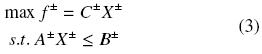Where X± is a vector of grey continuous and grey integer variables, A±{R±}m×n, B±{R±}1, (where R± stand for a set of grey numbers) and f± is a grey objective function. GMILP provides feasible ranges for the decision variables which are useful for decision makers to validate the generated alternatives. The solution of the grey binary variables have four possible presentation ([0,0], [1,1], [0,1], and [1,0]). They can be interpreted as grey decisions alternatives, reflecting potential system condition variations caused by the uncertainties. More information about Grey programming, as well as how to solve these problems, can be obtained from Huang and Moore (1993), Huang et al. (1992), Huang et al. (1993), Chen et al. (2004), and Liu and Lin (2006).

2.2 Heat exchanger network

Grey programming is easy to apply to HENs. The synthesis of HENs is one of the extensively studied problems in chemical engineering, where the objective is the generation of a network of heat exchangers either with minimum total utility consumption, minimum number of heat–transfer units, or minimum total cost. A critical review related to HENs synthesis was provided by Furman and Sahinidis (2002). The paper contains the chronological milestones in the development of the field. Another review related to important contribution of heat integration was presented by Moran and Agachi (2010). The paper contains a comprehensive review of the development through the years 1975 to 2008 of heat integration and HENs. The methods for the synthesis of HENs can be classified as heuristic–algorithmic–evolutionary and mathematical–programming (Grossmann et al., 1983). Usually in these methods nominal parameters values are used, e.g. constant values for source–heat–capacity–flowrates and temperatures. The problem of including uncertainties in these parameters in the synthesis of HENs has started to receive attention since 1980s. Several works have been published on flexibility of HENs including MILP transshipment model with the active set strategy to guarantee the desired HEN flexibility (Floudas and Grossmann, 1987; Konukman et al., 2002; Floudas and Grossmann, 1986; Chen and Hung, 2007).

Galli and Cerda (1991) presented a general algorithmic approach to the synthesis of flexible HENs which explicitly accounts for prespecified uncertainties in both supply temperatures and flowrates. Papalexandri and Pistikopoulos (1994a) developed a systematic framework for the synthesis and/or retrofit of flexible and structurally controllable heat exchanger networks. The proposed framework results in a network structure which features minimum annualized cost, while being flexible to operate within a specified range of uncertain stream flow rates, inlet temperatures, and/or heat transfer coefficients and structurally controllable. Also Papalexandri and Pistikopoulos (1994b) addressed the problem of how to synthesize mass and heat exchange processes, in which stream flowrates and their inlet compositions and temperatures may vary according to a set of discrete values. A multiperiod mixed integer nonlinear programming (MINLP) model was developed, where a total annualized cost is minimized by balancing capital investment cost to operating. A conceptual tool was presented to address the flexibility and operability objectives for HENs by Tantimuratha et al. (2001). The approach presented a screening model to accommodate the considerations ahead of design. The paper proposed a systematic algorithmic procedure that converges to flexible networks at the expense of only a minor set of additional constraints.

A simple way to synthesize HEN is the transshipment model, which solves the problem using sequential optimization. In this strategy the minimum utility consumption is determined first by means of a LP model, and based on these results the minimum number of matches is determined using a MILP model (Biegler, Grossmann and Westerberg, 1997). Two advantages of this procedure are: 1) its computational implementation is simple and can be solved with readily available software, and 2) the procedure is taught in many undergraduate–level courses and available in chemical process design texts. This means that this technology is within the knowledge of a chemical engineer.

3 Grey heat integration

3.1 Grey heat exchanger network

Pinch analysis is a methodology that allows the calculation of minimum hot and cold utilities consumptions in a process from the knowledge of some data of the hot and cold streams. This methodology is well known in the literature and involves the calculation of cascade composite hot and cold heat flows model (Biegler, Grossmann and Westerberg, 1997) based on initial and final temperatures of streams and its mean heat capacity flowrate. Let us denote Ch = {(T, Q)|Q = FCP(Tin –T), ToutTTin} as the Hohmann/Lockhart curve for a hot stream h, then the hot composite curve can be defined as CCH =Ch where Ω represents the composition of one curve based on the set of Ch, and H denotes the set of hot streams. A similar definition is possible for the cold streams, this is CC = {(T, Q)|Q = FCP(T – Tin), TinTTout} and CCc =Cc where C denotes the set of cold streams.

Then the grey hot Hohmann/Lockhart curve can be defined aswherei.e.,andBased in these definitions the grey hot composite curve is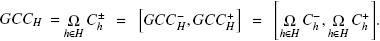In the same way, the grey cold Hohmann/Lockhart curve iswhere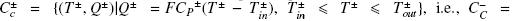andSimilarity the grey cold composite curve isAlso, it is possible to calculate the grey grand composite curve, which can be useful to analyze the heat integration problem under uncertain parameters. These definitions are general and can therefore be utilized in different methods, whether based on physical insights (Linnhoff, 1993; Linnhoff, 1990) or mathematical programming (Papoulias and Grossmann, 1993).

As an example, for process streams presented in Table 1, we can calculate the grey hot and cold composite curve (GCCH and GCCC) as it is shown in Fig. 1. Also Fig. 2 shows the grey grand composite curve. This problem is a modification of the example 16.1 presented by Biegler, Grossmann and Westerberg (1997), with steam available at 500ºC, cooling water 20–30ºC, and minimum grey recovery approach temperature of [10,10] ºC. This means that the approach temperature, for this example, is a deterministic number. For the calculation of grey minimum hot and cold utilities consumptions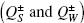we need to integrate the GCC+H with GCCC to determine QS and Q+W, and integrate GCCH with GCC+C to determine Q+S and QW. Also to determine the grey possible heat integration, Q±1, we need to integrate the GCC+H with GCC+C determine Q±1, and GCCH with GCCC to determine Q1.3.2 Grey transshipment model for HEN

The transshipment model for the calculation of the minimum utility consumption and the minimum number of units can be applied using GP to consider the uncertainty in the temperatures and the heat capacity flowrates. For example, the heat contents of the hot and cold processing streams can be calculated considering grey temperature intervals, which are based on the inlet and outlet temperatures and in the grey approach temperature. Table 2 shows the grey heat contents (MW) for our example. In this work the transshipment model proposed by Papoulias and Grossmann (1993) is used. The mathematical models are the same proposed in Papoulias and Grossmann, but grey numbers are used in heat contents in each interval. For example the GLP transshipment model for minimum utility consumption is:where the nomenclature is the same as indicated in the book of Biegler, Grossmann and Westerberg (1997). The right side of the Eq. (4) is calculated using the equation (5) to determine Qm–S and Qn+W and Eq. (6) to determine Qm+S and Qn–W.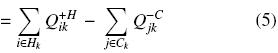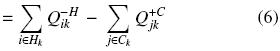Accordingly, the grey mathematical programming problem is solved in two steps, first eqs. (5) and (4) are used to determine Qm–S and Qn+W, and then eqs. (6) and (4) to determine Qm+S and Qn–W. All problems are LP, and therefore easy to calculate. Note that this is similar to calculate the "best" and "worst" cases for heat integration. After the calculation of grey minimum hot and cold utilities consumptions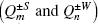we can calculate the grey fewest number of units in the network using the MILP transshipment model proposed by Papoulias and Grossmann (1993), but with GS which transforms the problem into a GMILP transshipment model. To define the subnetworks, a grey pinch point can be identified if a grey residual, Rk± has the lower bound equal to zero. In fact, the grey minimum utility cost with constrained matches and the grey minimum number of units models are similar to those given by Biegler, Grossmann and Westerberg (1997) but with grey numbers, and for that reason these equations are not given in this manuscript.

Table 3 contains the computational results obtained through the GLP and GMILP models. It should be pointed out that solution for the objective function values and decision variables are grey numbers. The GLP model gives grey numbers for the hot and cold utilities, with a grey pinch in interval 2 ( ). The GMILP model, for the minimum number of matches, gives a grey optima function equal to [5, 6]. Note that if the solution of grey binary number are [0,0] or [1,1] then the grey binary number is a deterministic number, meaning that the match cannot or can be adopted with certainty. This is the case for matches H1.C1 (above and below pinch), H2.C1, H2.C2 and H2.CW below pinch. On the other hand, the [0,1] and [1,0] solutions represent grey decisions and can provide decision alternatives according to the desired flexibility of the network. This is the case for matches ST.C1 above pinch, H1.C2 and H1.CW below pinch. Thus, decision alternatives can be generated by adjusting/shifting the grey number solutions within their solution intervals according to the operational conditions.

It is known that the MILP environment for the transshipment model can give a number of global solutions having the same number of units. This means that the results should be analyzed carefully, identifying all the solutions. This aspect is not discussed in this work.

4 Example

This example is a modification of example 16.2 given by Biegler, Grossmann and Westerberg (1997). It involves two hot and two cold streams, it also has two hot and one cold utility. The data are given in Table 4.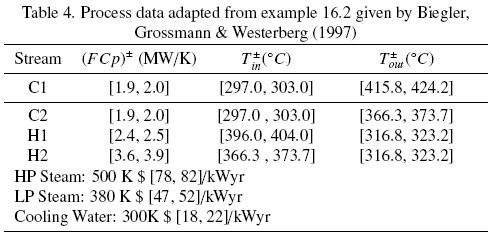The transshipment method for the grey minimum utility cost and grey minimum number of units were applied to this example. Solution for the objective function values and decision variables are grey numbers. The GLP model, for the grey minimum utility cost, gives cost [5.96, 8.17] M\$/yr, and the grey numbers for the hot and cold utilities. The values for the heat load of high pressure steam and low pressure steam are [42.2 , 78.6] MW and [25.0, 0.0] MW respectively. This means that the high–pressure steam is always necessary, but the low pressure steam under certain conditions may not be necessary. The values for the heat load of cooling water is [19.0, 147.9] MW. Table 5 gives the results.

The GMILP model, for the minimum number of matches, gives a grey optima function equal to [5, 7]. Note that if the values of grey binary number are [0,0] or [1,1] then the grey binary number is a deterministic number, meaning that the match cannot or can be adopted with certainty. This is the case for matches C1.H1, C2.H1, C1.H2 and C1.HPS. On the other hand, the [0,1] and [1,0] solutions represent grey decisions and can provide decision alternatives according to the desired flexibility of the network. This is the case for matches CW.H1, C2.H2, CW.H2 and C2.LPS. From these results we can conclude that between 5 and 7 units are required depending on the operation conditions. Of these, four matches should be included in the network with certainty. Then there are four other alternatives that should be assessed, for example using case studies or simulation, to make a final decision. Remember that the solution to the GMILP is not unique. These aspects are not discussed in this work.

Conclusions

Grey systems and grey programming methods have been applied to the synthesis of HENs. It improves upon other existing deterministic methods by allowing uncertain information to be directly considered into the synthesis/optimization process, and improves upon other non–deterministic methods by simplifying the mathematical problem. The results indicate that reasonable solutions have been generated for both continuous and binary variables.

The application of grey programming to HENs is novel; although in the literature, grey programming has been applied to design/synthesis problems in other engineering disciplines (waste treatment), there is not application to chemical engineering related problems in the literature. However, grey programming is very similar to interval programming, which is not new.

Although the application of GP was simple in the case of HENs, one of the questions that remain is the type of solution obtained. Indeed the representation of uncertainty with grey numbers gives us results that are also grey and therefore there is uncertainty in the solution. However, the methodology gives some light on part of the solution that is deterministic and where there is uncertainty.

Acknowledgments

The authors wish to thank CONICYT for support through Project PEL 81105010 and Fondecyt 1090406.

References

Biegler, L.T., Grossmann, I.E. and Westerberg, A.W. (1997). Systematic Methods of Chemical Process Design, Prentice Hall.         [ Links ]

Chen, C.L. and Hung, P.S. (2007). Synthesis of flexible heat exchange networks and mass exchange networks. Computers and Chemical Engineering 31, 1619–1632.         [ Links ]

Chen Z., Chen Q., Chen W. and Wang Y. (2004). Grey linear programming. Kybernetes 33 (2), 238–246.         [ Links ]

Floudas C.A. and Grossmann I.E. (1986). Automatic synthesis of optimum heat exchanger network configurations. AIChE Journal 32, 276.         [ Links ]

Floudas, C.A. and Grossmann, I.E. (1987). Synthesis of flexible heat exchanger networks with uncertain flowrates and temperatures. Computers and Chemical Engineering 11 (4), 319–336.         [ Links ]

Grossmann, I.E., Halemane, K.P. and Swaney, R.E. (1983). Optimization strategies for flexible chemical processes. Computers and Chemical Engineering 7 (4), 439–462.         [ Links ]

Galli, M.R. and Cerda, J. (1991). Synthesis of flexible heat–exchanger networks. 3. Temperature and flow–rate variations. Computers and Chemical Engineering 15 (1), 7–24        [ Links ]

Huang, G.H., Baetz B.W. and Patry G.G. (1992). A grey linear programming approach for municipal solid waste management planning under uncertainty. Civil Engineering Science 9, 319–335.         [ Links ]

Huang, G.H., Baetz B.W. and Patry G.G. (1993). A grey fuzzy linear programming approach for municipal solid waste management planning under uncertainty. Civil Engineering Science 10, 123–146.         [ Links ]

Huang G. and Moore R.D. (1993). Grey linear programming, its solving approach, and its applications. International Journal of Systems Science 24 (1), 159–172.         [ Links ]

Huang, G.H., Beatz, B.W. and Patry, G.G. (1995). Grey integer programming: An application to waste management planning under uncertainty. European Journal of Operational Research 83, 594–620.         [ Links ]

Konukman, A.E.S., Camurdan, M.C. and Akman, U. (2004). Simultaneous flexibility targeting and synthesis of minimum–utility heat–exchanger networks with superstructure–based MILP formulation. Chemical Engineering and Processing 41, 501–518.         [ Links ]

Liu, S. and Lin Y. (2006). Grey Information, Springer, USA.         [ Links ]

Linnhoff, B. (1993). Pinch analysis – a state–of–the–art overview. Chemical Engineering Research and Design 71 (a5), 503–522.         [ Links ]

Linnhoff, B. and Ahmad, S. (1990). Ahmad, S. Cost optimum heat–exchanger networks. 1. Minimum energy and capital using simple–models for capital costs. Computers and Chemical Engineering 14 (7), 729–750.         [ Links ]

Papoulias, S.A. and Grossmann, I.E. (1993). A structural optimization approach to process synthesis–II. Heat recovery networks. Computers and Chemical Engineering 8, 707.         [ Links ]

Papalexandri, K.P. and Pistikopoulos, E.N. (1994a). Synthesis and retrofit design of operable heat–exchanger networks. 1. Flexibility and structural controllability aspects. Industrial and Engineering Chemistry Research 33 (7), 1718–1737.         [ Links ]

Papalexandri, K.P. and Pistikopoulos, E.N. (1994b). A multiperiod MINLP model for the synthesis of flexible heat and mass–exchange networks. Computers and Chemical Engineering 18 (1112), 1125–1139.         [ Links ]

Tantimuratha, L., Asteris, G., Antonopoulos, D.K. and Kokossis, A.C. (2001). A conceptual programming approach for the design of flexible HENs. Computers and Chemical Engineering 25 (4–6), 887–892.         [ Links ]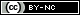Todo o conteúdo deste periódico, exceto onde está identificado, está licenciado sob uma Licença Creative Commons